Related Articles

# How to merge multiple DataFrames in R ?

• Last Updated : 30 Apr, 2021

In this article, we will discuss how to merge multiple dataframes in R Programming Language. Dataframes can be merged both row and column wise, we can merge the columns by using cbind() function and rows by using rbind() function

### Merging by Columns

cbind() is used to combine the dataframes by columns.

Syntax:

cbind(data1,data2,…………..,data n)

Parameters:

where data1 and data 2 are the data frames.

Example 1:

## R

 `# vector with student details``names1=``c``(``"sravan"``,``"bobby"``,``"ojaswi"``)`` ` `# vector with marks``marks1=``c``(90,89,78)`` ` `# pass these vectors to the ``# dataframe 1``data1=``data.frame``(names1=names1,marks1=marks1)``print``(data1)`` ` `# vector with student details``names2=``c``(``"gnanesh"``,``"rohith"``,``"divya"``)`` ` `# vector with marks``marks2=``c``(68,99,79)`` ` `# pass these vectors to the dataframe 2``data2=``data.frame``(names2=names2,marks2=marks2)``print``(data2)`` ` `print``("-------------------------------\``-------------------------------")`` ` `# merging these two dataframes using cbind``print``(``cbind``(data1,data2))`

Output: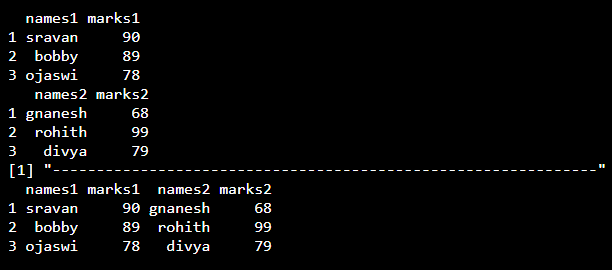Example 2:

We can also merge specific columns in each dataframe by using \$ operator we can access the dataframe column

Syntax:

dataframe_name\$columnname

## R

 `# vector with student details``names1=``c``(``"sravan"``,``"bobby"``,``"ojaswi"``)`` ` `# vector with marks``marks1=``c``(90,89,78)`` ` `# pass these vectors to the``# dataframe 1``data1=``data.frame``(names1=names1,marks1=marks1)``print``(data1)`` ` `# vector with student details``names2=``c``(``"gnanesh"``,``"rohith"``,``"divya"``)`` ` `# vector with marks``marks2=``c``(68,99,79)`` ` `# pass these vectors to the dataframe 2``data2=``data.frame``(names2=names2,marks2=marks2)``print``(data2)`` ` `print``("---------------------------------``\-----------------------------")`` ` `# merging these two data frames marks``# column using cbind``print``(``cbind``(data1\$marks1,data2\$marks2))`

Output: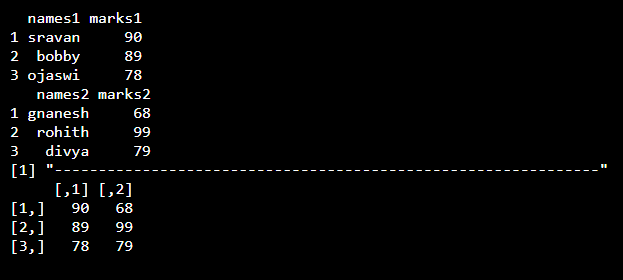Example 3:

## R

 `# vector with student details``names1=``c``(``"sravan"``,``"bobby"``,``"ojaswi"``)`` ` `# vector with marks``marks1=``c``(90,89,78)`` ` `# pass these vectors to the ``# dataframe 1``data1=``data.frame``(names1=names1,marks1=marks1)``print``(data1)`` ` `# vector with student details``names2=``c``(``"gnanesh"``,``"rohith"``,``"divya"``)`` ` `# vector with marks``marks2=``c``(68,99,79)`` ` `# pass these vectors to the dataframe 2``data2=``data.frame``(names2=names2,marks2=marks2)``print``(data2)`` ` `# vector with student details``names3=``c``(``"bhavya"``,``"harsha"``,``"navya"``)`` ` `# vector with marks``marks3=``c``(68,99,79)`` ` `# pass these vectors to the dataframe 3``data3=``data.frame``(names3=names3,marks3=marks3)``print``(data3)`` ` `print``("-----------------------------------\``---------------------------")`` ` `# merging these three data frames``print``(``cbind``(data1,data2,data3))`

Output: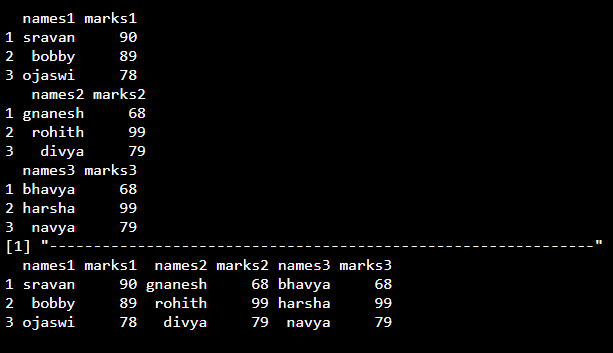### Merging by Rows

We can merge the rows between the dataframes using rbind() function.

Syntax:

rbind(dataframe1,dataframe2)

Example 1:

## R

 `# create vectors``x=``c``(1,2,3,4,3,4,5)``y=``c``(4,5,6,7,2,3,4)`` ` `# pass these vectors to the ``# input of dataframe1(a)``a=``data.frame``(x,y)`` ` `# create vectors``x=``c``(10,20,30,40,50,60,70)``y=``c``(40,50,60,40,50,60,70)`` ` `# pass these vectors to the ``# input of dataframe1(a)``b=``data.frame``(x,y)`` ` `# apply rbind function to ``# merge rows``print``(``rbind``(a,b))`

Output: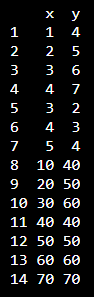Example 2:

## R

 `# create vectors``x=``c``(1,2,3,4,3,4,5)``y=``c``(4,5,6,7,2,3,4)`` ` `# pass these vectors to the ``# input of dataframe1(a)``a=``data.frame``(x,y)`` ` `# display dataframe``print``(a)``print``(``"---------------------"``)`` ` `# create vectors``x=``c``(``"sravan"``,``"bobby"``)``y=``c``(``"Eswar"``,``"sai"``)`` ` `# pass these vectors to the ``# input of dataframe1(a)``b=``data.frame``(x,y)`` ` `# display dataframe``print``(b)`` ` `# apply rbind function to merge rows``print``(``rbind``(a,b))`

Output: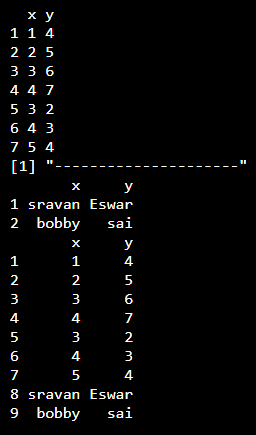My Personal Notes arrow_drop_up